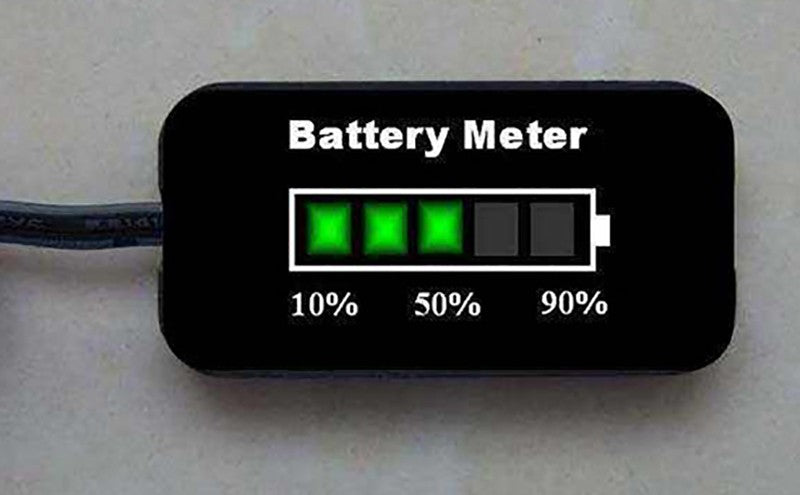## 1.What is battery state of charge monitoring technology

The monitoring technology of battery state of charge is a technique used to predict battery capacity when all systems are running or idle.

Expression of battery capacity：

- The percentage

- Running time

- To the time when the battery is exhausted/full

- Milliampere hour (mAh)

- Watt hour (Wh)

- Call time and limit time

We monitor the battery state of charge to report the capacity of the battery, but this technology can also provide the health status of the battery and the full capacity of the battery. Other data generally available to reflect battery health and safety diagnosis are ① health status ② full charge capacity

## 2.Basic knowledge of battery chemical composition related to battery state of charge

• Discharge curve of lithium ion battery

The figure below depicts lithium ion battery discharge curve.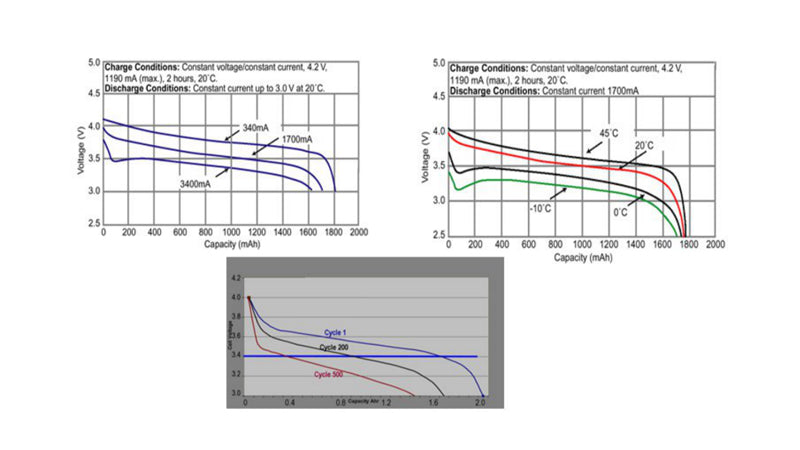The three graphs here are the discharge curves of lithium ion batteries measured under different conditions. The cut-off voltage changes with discharge rate, temperature and aging conditions to provide as long a running time as possible. From these figures, we can first see that the battery voltage will drop quickly under the condition of low current at room temperature. Although the lowest voltage supported by the system can reach 2.5V, the voltage will drop quickly. In order to avoid data loss or sudden interruption of the circuit loading files caused by sudden shutdown, customer application systems generally tend to set the reference point of the battery to 340mA with a minimum capacity. If at low temperature or high current conditions, or in the case of very old battery, still use zero as a reference point of battery state of charge, it will cause a large reduction in the available capacity of the battery. As you can see from these curves, in the case of high current, the discharge curve is almost there from the beginning, and it's similar in the case of aging or low temperature. Therefore, if fixed as a reference point with zero capacity, the reported capacity will be reduced in the case of low temperature or high current or near aging. To avoid this situation, the battery capacity needs to be adjusted according to the temperature, discharge rate and aging degree of the battery.The high-current discharge capacity of lithium-ion batteries can be adjusted over a wide range by using thicker or thinner layers of active materials. A thinner layer of active material means that the battery has a higher capacity to discharge large current, but a lower energy density. The standard 18650 cylindrical battery used in notebook computers is designed for maximum C-rate discharge. However, some batteries are rated for 10C discharge (for portable power tools) and even 60C discharge (for backup power/regenerative braking in gas-electric hybrid vehicles).

The high current discharge capacity is severely reduced at low temperatures below 0℃ due to the low conductivity of organic electrolytes. Electrolyte conductivity varies, so it is important to check the manufacturer's data on low temperature discharges.

• Battery chemical capacity (Qmax)

About the monitoring technology of battery state of charge, there is a more important concept, is the chemical capacity of the battery, Qmax.

In this picture, the red curve intersects the x-coordinate of Qmax.

This curve is measured when the load current is zero, because the load current must be small enough to measure Qmax. Theoretically, Qmax refers to the capacity that can be released when the current approaches zero. But in practice, engineering technology uses a very small current to measure Qmax, and here we use the current. So why is it?

In terms of battery state of charge management, C refers to the discharge rate of the battery. In fact, 1C refers to 1C if the capacity of the battery is 2200mAh and the current is 2200mA. Therefore, it is the current required to completely empty a battery within one hour. Therefore, the discharge current of a 2200mAh battery is 2200mA, and the discharge current of that battery is 1100mA.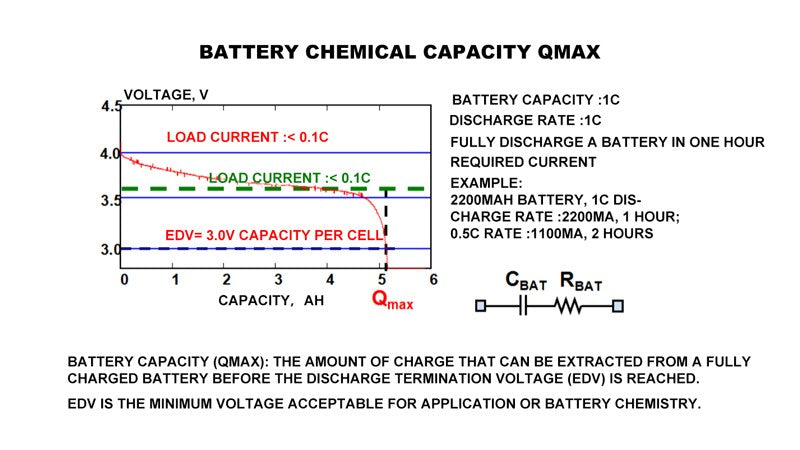The EDV in this picture refers to the lowest voltage that the system or battery itself can support.

In portable applications, the main question about battery functionality is "How long will it last?" This is determined by the amount of active material, its specific capacity and voltage characteristics. When a battery discharges, its voltage drops gradually until it reaches the minimum voltage acceptable to the device, at which the battery will be damaged if it continues discharging. By integrating the charge transferred during the discharge process, we can measure the capacity Qmax that can be discharged before EDV is reached. The voltage curve of a low rate lithium ion battery during discharge is illustrated above.

• Available capacity (Quse)

There is also a corresponding capacity about battery state of charge which is the available capacity. Because just now is talking about the chemical capacity of the battery, the chemical capacity of the battery is measured when the current is very small, it is more determined by the characteristics of the battery itself. The actual, in the process of battery capacity is not so much to be able to put it, in the actual use process, because there is a certain discharge current, so the discharge curve will be lower than the open circuit voltage curve. You can see this curve, due to the existence of battery internal resistance, actual discharge curve is the blue curve. The blue curve and the red curve correspond to the value of Quse, which actually refers to the available capacity of the battery. In this curve, we find that the position of the curve is moved down due to the existence of the internal resistance of the battery, so the discharge termination voltage will be reached earlier, that is, EDV will be reached earlier. So Quse is generally less than Qmax.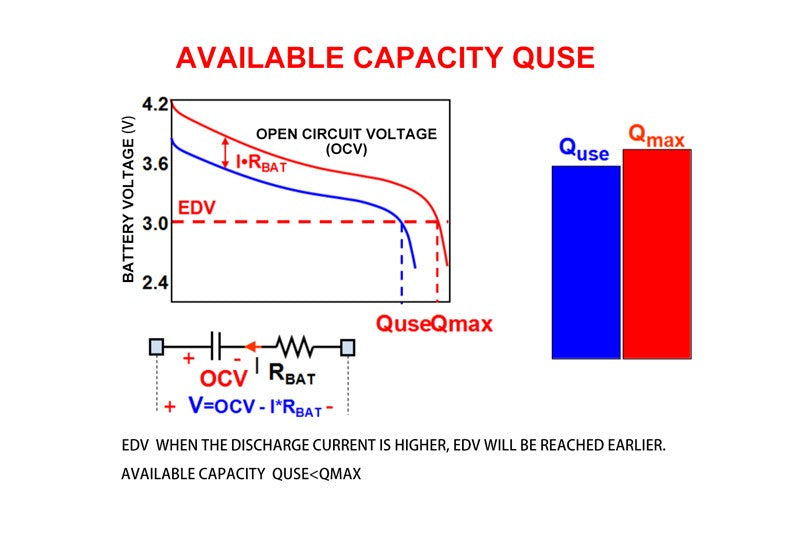We can also see from this curve that the higher the current, the lower the Quse will be. In this curve, I*Rbat refers to the drop in battery terminal voltage due to the presence of internal resistance.

• Battery resistance
• Impedance = F (T, SOC and aging)
• The resistance will double after 100 charges and discharges
• Resistance deviation between batteries is 10-15%
• Battery resistance can vary by 10-15% from manufacturer to manufacturer

The internal resistance of battery has a very important effect on the monitoring of battery voltage. The basic formula can be used to express the influence of battery internal resistance on battery state of charge monitoring:

V=Vocv-I*Rbat

In this formula, Vocv refers to the open circuit voltage of the battery, I refers to the charge-discharge current, Rbat refers to the internal resistance of the battery, and V refers to the terminal voltage of the battery.

The impedance of a battery is actually influenced by many factors, such as ambient temperature, the percentage of capacity of the battery, and the degree of aging of the battery. It's a very complicated function of these variables. Now it is very difficult to get the specific expression of this function, so the actual measurement method is often used to get the impedance, that is, the difference table method to get the impedance. As a rule of thumb, the internal resistance of the battery usually doubles after 100 charges and discharges. The deviation between the same batch of batteries is well controlled and can be controlled at about 10 to 15%. The deviation between the internal resistance of batteries produced by different battery manufacturers is often larger. So the battery internal resistance is difficult to control its deviation in the production of a small variable, the battery internal resistance is a very difficult to control the variable, is also a very important variable.

• Battery state of charge (SOC)

So battery state of charge is actually the percentage of capacity, which is the percentage of capacity in the corner of the screen when you're using your phone or tablet a lot, and the percentage of capacity is how much power is left in the battery between a certain state and when it's empty. So obviously for a full charge battery, the battery state of charge equal to 1; The percentage of voltage applied to a completely empty cell is equal to zero. So the formula for the percentage of voltage is equal to Q (the remaining capacity at state A) on this curve divided by the chemical capacity of the battery Qmax.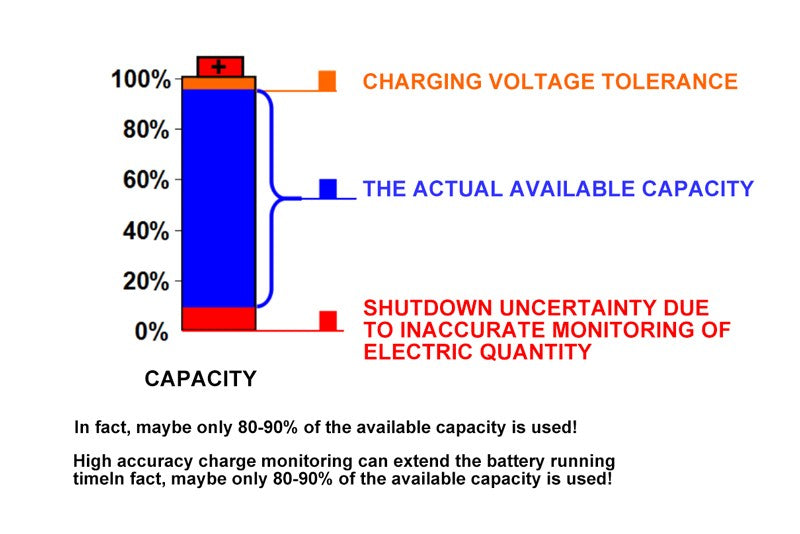One of the concepts that corresponds to the battery state of charge that we usually use is DOD, which is the Depth Of Discharge. So obviously when the battery state of charge or the capacity percentage is 1, then the discharge depth should be 0; Conversely, when the percentage of capacity is 0, the discharge depth should be 1.

DOD is a concept that we often come across, DOD is actually opposite to SOC, and DOD is actually the same thing, which is how much charge is left in the battery, or how much charge has been discharged since the battery was fully charged, in terms of this degree.

• Resistance is related to temperature and DOD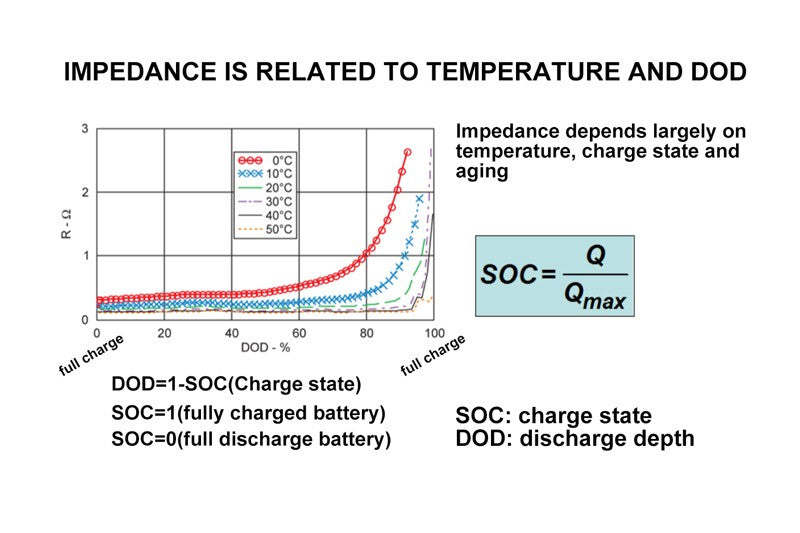Therefore, the impedance of the battery is greatly affected by temperature and percentage of capacity, which can also be expressed by the discharge depth just mentioned, namely DOD. From this curve, we can see some basic trends. From the graph, we can see that the higher the discharge percentage and the higher the discharge depth, the greater the internal resistance of the battery, because the vertical axis on this curve refers to the internal resistance of the battery, which is in ohms; The abscissa is the percentage of discharge, DOD. The curves in different colors show the data measured at different temperatures. Obviously, at the same temperature, the greater the percentage of discharge, that is, the deeper the discharge, the greater the internal resistance of the battery. So we can also see in this figure that under the same DOD, that is, under the same percentage of capacity, the lower the temperature, the greater the internal resistance of the battery. That's a basic concept.

• Impedance and capacity change with aging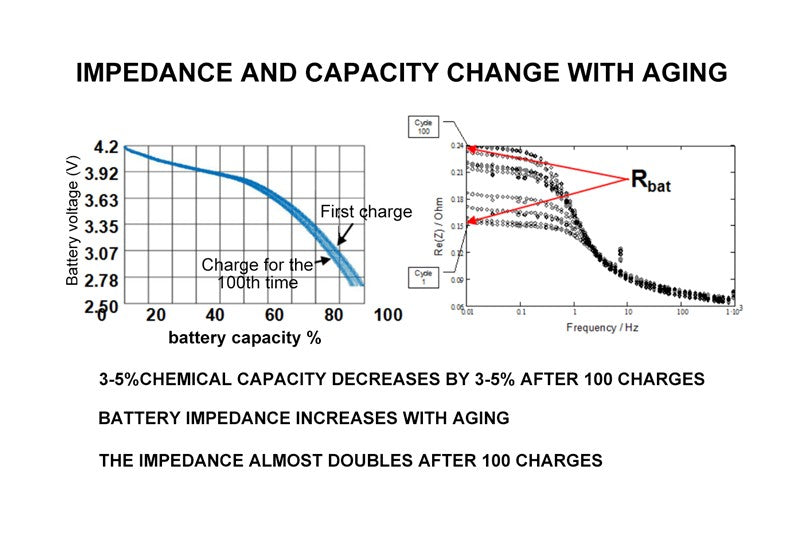The internal resistance of the battery is related to temperature and percentage of capacity, and another factor that affects the life of the battery is the aging degree of the battery. After 100 times of redischarge, the chemical capacity of the battery will be reduced by 3~5%. This capacity reduction is not very significant, but its impedance change is more significant. After 100 times of charge and discharge, the impedance can be increased almost 1 times. As can be seen from these two pictures, the one on the left is a picture of the discharge curves of the first and the 100th times drawn together. It can be seen from this picture that the increase of discharge times has not great influence on the reduction of capacity. However, the increase of discharge rate has a great impact on the internal resistance. The chart on the right shows the relationship between the internal resistance of the battery and the increase of discharge times. There are many curves in this chart.

In this picture, we can see that in the case of low frequency, the curve is on the bottom of the picture is the first curve measured under different frequencies, the curve on the top is the 100th time under different frequency measurement of battery internal resistance curve, the two curves is the ordinate intersection point of the basic difference between the twice, so after 100 cycles, The internal resistance of the battery has doubled. Here, the x-coordinate of internal resistance is frequency, indicating that at a very low frequency, the change of internal resistance is significant as the number of cycles increases, but conversely, as the frequency increases, for example: When the test load variation frequency is increased to 1KHZ, the change of internal resistance can be ignored, and you can see that all these curves converge to the same point. So what is the impedance that actually has a big impact on our battery state of charge detection?

Is the impedance in the case of low frequency or DC, so we should look at the two intersection points of the curve and the ordinate on the right. From this intersection point, we can see that the number of cycles affects the dc internal resistance of the battery.

• The impedance difference of the new battery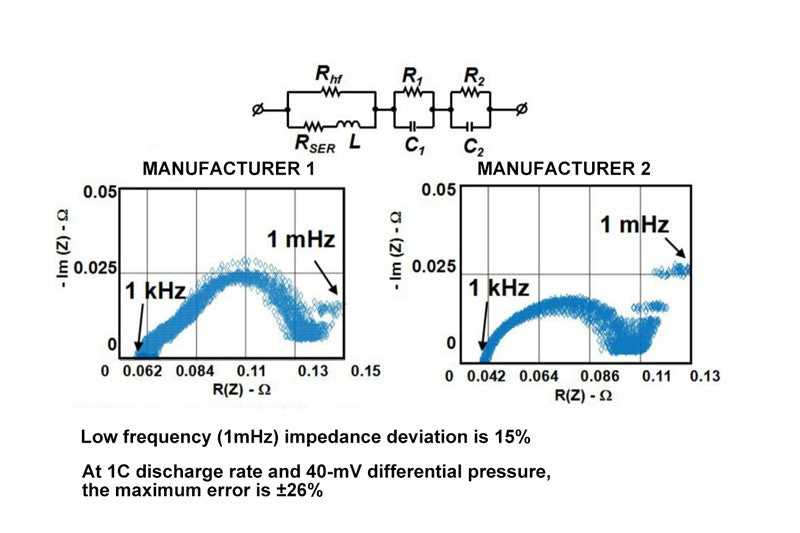This graph shows the difference in impedance of a new battery. The process structure of the battery is stacked or rolled up layer by layer, so the positive and negative electrodes of the battery can be seen from the outside, the characteristics of capacitance, resistance, and inductance. So the battery, if you want to measure the impedance of the it, impedance can be divided into real and imaginary part. In this picture, we use an alternating load to determine the battery internal resistance, the battery change frequency, which is the change of the load current frequency, from 1 KHZ to 1 MHZ, we often come into contact with the concept of 1 KHZ, is 1 second change 1000 times; 1mHZ is a change in 1000 seconds, the change frequency is quite slow, that is to say, the measurement is actually a DC impedance.

In this two picture, you can see, the dc resistance is as low frequency monotonous linear increase, but it has a change trend of ac impedance, is small at the beginning, subsequent slowly become larger, then small, then change, this is because the battery is internal capacitance and inductance due to these factor synthesis influence. But the DC impedance increases monotonously, and as the frequency decreases, the DC impedance becomes larger and larger. Then for the monitoring technology of battery state of charge manufacturers usually use, we are concerned about the 1 MHZ dc resistance, we can see from this picture, 1 MHZ cases, battery impedance deviation is about 15%, the 15% or so of the impedance deviation will cause if it is 1 c discharge current, voltage of the battery and the open circuit voltage differential 40 mv low temperatures, If you use an algorithm to judge capacity based on voltage, it will cause a capacity error of about 26%.

• Remaining battery capacity (RM)

Here is the remaining capacity of the battery. Remaining capacity refers to the current state of the battery to the EDV, which is the termination voltage. In the figure, the current state A discharges at a given current current. When it discharges to the given point, the corresponding remaining capacity is marked RM1 from the figure. So if, in state A, this curve is a little bit lower than the open circuit voltage and a little bit lower than the RM1 corresponding to the small current discharge, then the remaining capacity is RM2. The difference between the discharge curves of RM2 and RM1 is that the discharge current is different. The higher the discharge current is, the lower the curve position is, and the smaller the remaining capacity is. Therefore, the remaining capacity of the battery is related to the discharge rate. Some users in the process of the actual use of the battery in the case of discharge, will find that the capacity of the battery from less to more, he felt an understanding, actually can explain here, this situation is caused by the change of discharge current, when we see battery capacity change from few to many, this is usually caused by discharge current suddenly become smaller. Because the capacity that the battery can release is not the same at different current, when the discharge current becomes smaller, the capacity that it can release can increase.

## 3.Conclusion

Let's just briefly review the concepts relate to the battery state of charge we introduced

Qmax = Chemical capacity of battery (no load)

Quse = Available capacity (load related)

Battery resistance and load produce an I-R voltage drop

SOC = state of charge (%, depends on OCV)

RM = Remaining capacity (depending on load)

Battery aging affects impedance and capacity.Qmax refers to the chemical capacity of the battery. The value of this capacity is load independent. This is the capacity that can be released by a battery under very low load current, usually expressed in mAh.

Quse refers to the available capacity of the battery. This capacity is related to the load. Under different loads, the available capacity of the battery is different. The higher the load current is, the lower the available capacity of the battery is.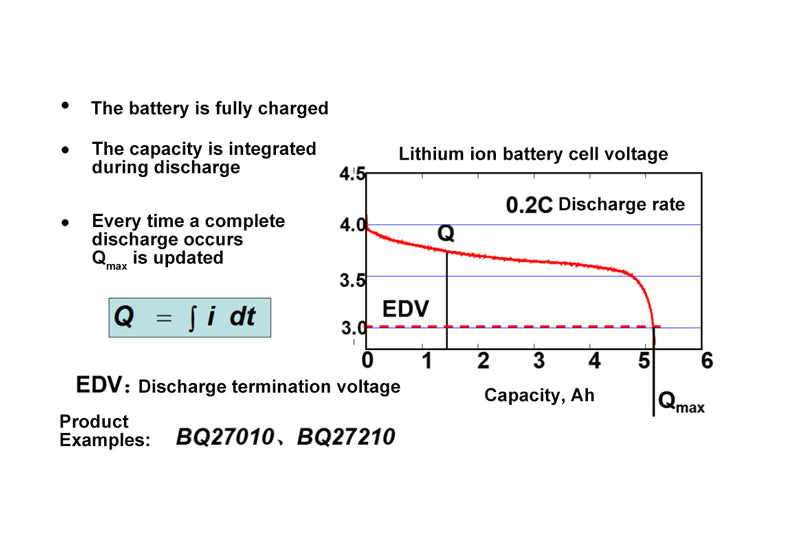Why does Quse and Qmax make such a difference? This is mainly due to the fact that the internal resistance of the battery and the load directly produce a voltage drop in the electromotive force and terminal voltage of the battery. There is also the concept of the battery capacity percentage, or  battery state of charge which is expressed in units of %. This % is actually the remaining capacity of the battery divided by the chemical capacity of the battery.

The remaining capacity is called RM. The size of RM depends on the load. The larger the load is, the smaller the remaining capacity is in the same state.There is also the notion that aging affects the impedance and capacity of the battery. The effect of aging on the impedance is obvious, and the impedance will increase twice after 100 cycles. The effect of aging on capacity is not as obvious as impedance, but there is a drop of 3 to 5% after 100 cycles.